Processing ......FreeComputerBooks.com Links to Free Computer, Mathematics, Technical Books all over the World

Probability Theory: The Logic of Science
🌠 Top Free Mathematics Books - 100% Free or Open Source!
• Title Probability Theory: The Logic of Science
• Author(s) E. T. Jaynes
• Publisher: Cambridge University Press (June 9, 2003)
• Hardcover 758 pages
• eBook HTML, PostScript, and PDF Files
• Language: English
• ISBN-10/ASIN: 0521592712
• ISBN-13: 978-0521592710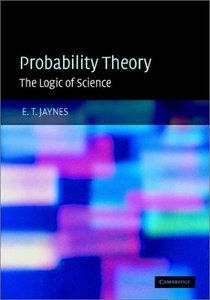Book Description

Going beyond the conventional mathematics of probability theory, this study views the subject in a wider context. It discusses new results, along with applications of probability theory to a variety of problems. The book contains many exercises and is suitable for use as a textbook on graduate-level courses involving data analysis. Aimed at readers already familiar with applied mathematics at an advanced undergraduate level or higher, it is of interest to scientists concerned with inference from incomplete information.

This is a book written by a scientist for scientists. As such it is to be welcomed. The reader will certainly find things with which he disagrees, but he will also find much that will cause him to think deeply not only on his usual practice by also on statistics and probability in general.

• Mark Pinsky is Professor of Mathematics at Northwestern University, where he has been since 1968, following a two-year postdoctoral position at Stanford. He completed his PhD at MIT in 1966, under the direction of Henry McKean. His published work includes 125 research papers and 10 books, including several conference proceedings and textbooks.
• Bjorn Birnir is a Professor of Mathematics at the University of California at Santa Barbara (UCSB). He served as the UCSB coordinator for nonlinear science from 1985-1990. He is currently the director of the Center for Complex and Nonlinear Science at UC Santa Barbara.
Reviews, Ratings, and Recommendations: Related Book Categories: Read and Download Links:Similar Books:
•Introduction to Probability, Statistics, and Random Processes

This book introduces students to probability, statistics, and stochastic processes. It provides a clear and intuitive approach to these topics while maintaining mathematical accuracy. The book contains a large number of solved exercises.

•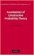Foundations of Constructive Probability Theory (Yuen-Kwok Chan)

This book provides a systematic and general theory of probability within the framework of Constructive Mathematics. It can serve as a parallel introduction into constructive mathematics and rigorous probability theory.

•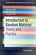Introduction to Random Matrices: Theory and Practice

This is a book for absolute beginners. The aim is to provide a truly accessible introductory account of Random Matrix theory. Most chapters are accompanied by MATLAB codes to guide readers through the numerical check of most analytical results.

•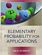Elementary Probability for Applications (Rick Durrett)

This clear and lively introduction to probability theory concentrates on the results that are the most useful for applications, including combinatorial probability and Markov chains. Concise and focused, it is designed for students familiar with basic calculus.

•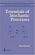Essentials of Stochastic Processes (Rick Durrett)

Stochastic processes have become important for many fields, including mathematical finance and engineering. Written by one of the worlds leading probabilists, this book presents recent results previously available only in specialized monographs.

•Introduction to Probability (Charles M. Grinstead, et al)

The book is a beautiful introduction to probability theory at the beginning level. The book contains a lot of examples and an easy development of theory without any sacrifice of rigor, keeping the abstraction to a minimal level.

•Probability Theory: The Logic of Science (E. T. Jaynes)

Going beyond the conventional mathematics of probability theory, this study views the subject in a wider context. It discusses new results, along with applications of probability theory to a variety of problems.

•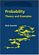Probability: Theory and Examples (Rick Durrett)

This lively introduction to measure-theoretic probability theory covers laws of large numbers, central limit theorems, random walks, martingales, Markov chains, ergodic theorems, and Brownian motion.

•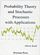Probability Theory and Stochastic Processes with Applications

This book provides an introduction to probability theory and discrete and continuous stochastic processes and its applications. It has a unique approach that provides a broad and wide introduction into the fascinating area of probability theory.

Book Categories
 :All CategoriesRecent BooksMiscellaneous BooksComputer LanguagesComputer ScienceData Science/DatabasesElectrical EngineeringJava and Java EE (J2EE)Linux and UnixMathematicsMicrosoft and .NETMobile ComputingNetworking and CommunicationsSoftware EngineeringSpecial TopicsWeb Programming
Other Categories